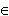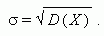Characteristics of random variables

Mathematical expectation. Properties of mathematical expectation.

Variance. Properties of variance. Root-mean-square deviation.

Mathematical expectation of a discrete random variable X , which receives a finite number of values õ i with probabilities ð i , is called the sum:

Ì ( Õ ) = õ 1 · ð 1 + õ 2 · ð 2 + õ 3 · ð 3 + ... + õ n · ð n .

Properties of mathematical expectation:

1) Ì ( ñ · Õ ) = ñ · Ì ( Õ ) , cR ,

2) Ì ( Õ + Y ) = Ì ( Õ ) + Ì ( Y ) , Õ , YÅ ,

3) Ì ( Õ · Y ) = Ì ( Õ ) · Ì ( Y )   for independent random variables Õ and Y .

Variance of a random variable X is called the number:

D ( Õ ) = Ì { [ Õ Ì ( Õ )] 2 }= Ì ( Õ 2 ) – [ Ì ( Õ )] 2 .

Properties of variance:

1) D ( ñ · Õ ) = ñ 2 · D ( Õ ) , cR ,

2) D ( Õ + Y ) = D ( Õ ) + D ( Y )   for independent random variables Õ and Y .

Root-mean-square deviation: